• python实现描述性统计、频数分布图、正态分布检验、概率密度曲线拟合 from scipy.stats import chi2 # 卡方分布 from scipy.stats import norm # 正态分布 from scipy.stats import t # t分布 from scip...

描述性统计
频数分布图
正态分布检验
概率密度曲线拟合

#单个项目数据分析
#单个项目描述性统计
from scipy.stats import chi2                 # 卡方分布
from scipy.stats import norm                 # 正态分布
from scipy.stats import t                    # t分布
from scipy.stats import f                    # F分布
import matplotlib.pyplot as plt
import numpy as np
import pandas as pd
import scipy.stats as stats
from scipy.stats import chi2_contingency     # 列联表分析

# rvs: Random Variates
# pdf: Probability Density Function                         概率密度函数
# cdf: Cumulative Distribution Function                     概率密度函数的积分函数
# sf: Survival Function (1-CDF)
# ppf: Percent Point Function (Inverse of CDF)              百分点函数，概率密度函数的积分值
# isf: Inverse Survival Function (Inverse of SF)
# stats: Return mean, variance, (Fisher’s) skew, or (Fisher’s) kurtosis
# moment: non-central moments of the distribution

# ppf以概率的形式，查询函数值-----------类似分布临界表

# example -------------------------------------------------------- 对连续数据进行正态拟合
plt.figure()
train = pd.read_csv("test.csv")
train_Age = train.dropna(subset=['p1'])
M_S = stats.norm.fit(train_Age['p1'])                            # 正态拟合的平均值与标准差

plt.hist(train_Age['p1'],bins=30, normed=1, facecolor='blue', alpha=0.5) # 原本的概率直方图
train_Age['p1'].plot(kind='kde',secondary_y=True)                                 # 原本的概率密度分布图

normalDistribution = stats.norm(M_S, M_S)                   # 绘制拟合的正态分布图

#x = np.linspace(normalDistribution.ppf(0.01), normalDistribution.ppf(0.99), 100)
x = np.linspace(normalDistribution.ppf(0.01), normalDistribution.ppf(0.99), 100)
plt.plot(x, normalDistribution.pdf(x), c='orange')
plt.xlabel('reqirement time')
plt.title('reqirement time on NormalDistribution', size=20)
plt.legend(['Origin', 'NormDistribution'])

from scipy import integrate
for n in range(0,400,1):
x=np.linspace(0,n,1000)
y=normalDistribution.pdf(x)
p=integrate.trapz(y, x)
if p>0.8:
print (n)
break

#多个项目批量输出分析结果
from scipy.stats import chi2                 # 卡方分布
from scipy.stats import norm                 # 正态分布
from scipy.stats import t                    # t分布
from scipy.stats import f                    # F分布
import matplotlib.pyplot as plt
import numpy as np
import pandas as pd
import scipy.stats as stats
from scipy.stats import chi2_contingency     # 列联表分析
from scipy import integrate
from scipy.stats import kstest               #检验正态分布
from statsmodels.stats.diagnostic import lillifors
# rvs: Random Variates
# pdf: Probability Density Function                         概率密度函数
# cdf: Cumulative Distribution Function                     概率密度函数的积分函数
# sf: Survival Function (1-CDF)
# ppf: Percent Point Function (Inverse of CDF)              百分点函数，概率密度函数的积分值
# isf: Inverse Survival Function (Inverse of SF)
# stats: Return mean, variance, (Fisher’s) skew, or (Fisher’s) kurtosis
# moment: non-central moments of the distribution

# ppf以概率的形式，查询函数值-----------类似分布临界表

train = pd.read_csv("test.csv")
#设置画布大小
fig = plt.figure(figsize=(16, 16))
#循环：依次计算p1~p46

#projects_index = ['p1', 'p2', 'p3', 'p4','p5', 'p6', 'p7', 'p8','p9', 'p10','p11', 'p12', 'p13', 'p14','p15', 'p16', 'p17', 'p18','p19', 'p20', 'p21', 'p22', 'p23', 'p24','p25', 'p26', 'p27', 'p28','p29', 'p30', 'p31', 'p32', 'p33', 'p34','p35', 'p36', 'p37', 'p38','p39', 'p40','p41', 'p42', 'p43', 'p44','p45', 'p46' ]
projects_index = ['p1', 'p2', 'p3', 'p4','p5', 'p6', 'p7', 'p8','p9', 'p10',
'p11', 'p12', 'p14', 'p15', 'p16', 'p17', 'p18','p19', 'p20',
'p21', 'p22', 'p23', 'p24','p25', 'p26', 'p27', 'p28','p29', 'p30',
'p31', 'p32', 'p33', 'p34','p35',  'p36', 'p38', 'p40',
'p41', 'p42', 'p43', 'p45', 'p46' ]
#projects_index = ['p1','p2','p3','p4','p5', 'p6', 'p7', 'p8']
count=0

#计算统计量
#创建一个空的Dataframe
Req_Leadtime = pd.DataFrame(columns=('项目名称','有效需求总数','平均值','中位数','众数','标准差','p值','需求交付周期阈值'))

for index in projects_index:
count+=1                                                        #计数
#print(count)
print(index)
train_time = train.dropna(subset=[index])
# 正态拟合的平均值与标准差
M_S = stats.norm.fit(train_time[index])

# 0-画子图
#ax = fig.add_subplot(14, 3, count)

#1-依次作图保存

# 原本的概率直方图
plt.hist(train_time[index],bins=30, normed=1, facecolor='blue', alpha=0.5)
# 原本的概率密度分布图
train_time[index].plot(kind='kde',secondary_y=True)
# 绘制拟合的正态分布图
normalDistribution = stats.norm(M_S, M_S)
x = np.linspace(normalDistribution.ppf(0.01), normalDistribution.ppf(0.99), 100)
plt.plot(x, normalDistribution.pdf(x), c='orange')

#计算平均值
avg_leadtime = np.mean(train_time[index])
#计算中位数
med_leadtime = np.median(train_time[index])
#计算众数
mode_leadtime = stats.mode(train_time[index])
#计算标准差
#std_leadtime = pd.std(train_time[index])
std_leadtime = np.std(train_time[index], ddof=1)
#检验正态分布，from scipy.stats import kstest，p为正态检验p值，>0.05
#rvs：待检验的数据
#cdf：检验方法，这里我们设置为‘norm’，即正态性检验
#alternative：默认为双尾检验，可以设置为‘less’或‘greater’作单尾检验
#q,p = kstest(train_time[index], 'norm',(avg_leadtime,std_leadtime))
#print(q,p)
#p = stats.shapiro(train_time[index])
if len(train_time[index])<8:
p = -1
else:
if len(train_time[index])<50:
q,p = stats.normaltest(train_time[index])
else:
if 50<=(len(train_time[index]))<=300:
#p = lillifors(train_time[index])
q,p = kstest(train_time[index], 'norm')
else:
q,p = kstest(train_time[index], 'norm')

print(p)

#计算80%需求交付周期
for n in range(0,400,1):
x=np.linspace(0,n,1000)
y=normalDistribution.pdf(x)
h=integrate.trapz(y, x)

if h>0.8:
print (n)
break

#计算统计量
#将计算结果逐行插入Req_Leadtime,注意变量要用[]括起来,同时ignore_index=True，否则会报错，ValueError: If using all scalar values, you must pass an index
valid_req_counts = len(train_time[index])
req_leadtime_avg = avg_leadtime
req_leadtime_med = med_leadtime
req_leadtime_mode = mode_leadtime
req_leadtime_std = std_leadtime
req_leadtime_p = p
req_leadtime_ref = n
Req_Leadtime = Req_Leadtime.append(pd.DataFrame({'项目名称':[index],
'有效需求总数':[valid_req_counts],
'平均值':[req_leadtime_avg],
'中位数':[req_leadtime_med],
'众数':[req_leadtime_mode],
'标准差':[req_leadtime_std],
'p值':[req_leadtime_p],
'需求交付周期阈值':[req_leadtime_ref]}),ignore_index=True)
print(req_leadtime_p,p)

plt.xlabel('Req leadtime')
plt.title("(%s) Req_leadtime on NormalDistribution, p = %10.3
f, Req_leadtime_req = %d" %(index,p,n),fontsize=20)
plt.legend(['Origin', 'NormDistribution'])

# 0-自动调整子图的间距
#plt.tight_layout()

#保存图片
plt.savefig("image/'" + index + "'.png")
#单独输出图片，需要清空画布
plt.clf()

#输出统计量
print(Req_Leadtime)
#输出到txt中
Req_Leadtime.to_excel('Req_Leadtime.xlsx', encoding='utf-8', index=True, header=True)



展开全文数据分析
• 最近的工作中有一个需求就是，给我一批历史的数据，需要我基于统计分布模型来去拟合一下原始的数据，挖掘出来数据最有可能的统计分布是怎么样的，为后面的参数区间计算或者是概率值计算提供一个指导。 下面是我手...
       最近的工作中有一个需求就是，给我一批历史的数据，需要我基于统计分布模型来去拟合一下原始的数据，挖掘出来数据最有可能的统计分布是怎么样的，为后面的参数区间计算或者是概率值计算提供一个指导。

下面是我手中数据的概率分布情况：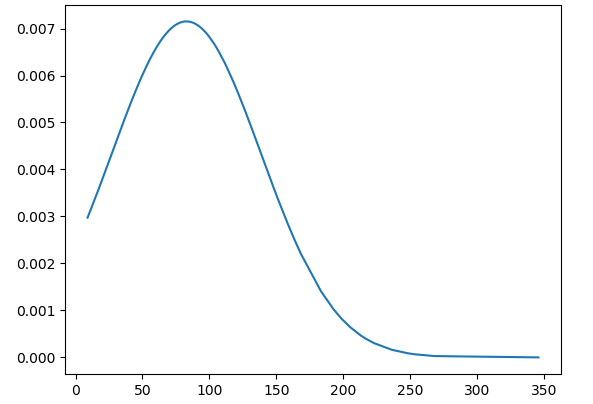从上面的概率分布函数曲线来看并不是一个正态分布的模型，所以我们就需要来去找别的统计模型来进行拟合分析了，标准模型比如：正态分布模型、F模型等都是为了简化实际中的计算出现的，基于上述的分布形状，这里基于scipy来寻找匹配性最好的模型。

借助于自己残存的一点点统计模型知识，上述的数据分布与我印象中的F分布比较相似，这里先来看一下F分布：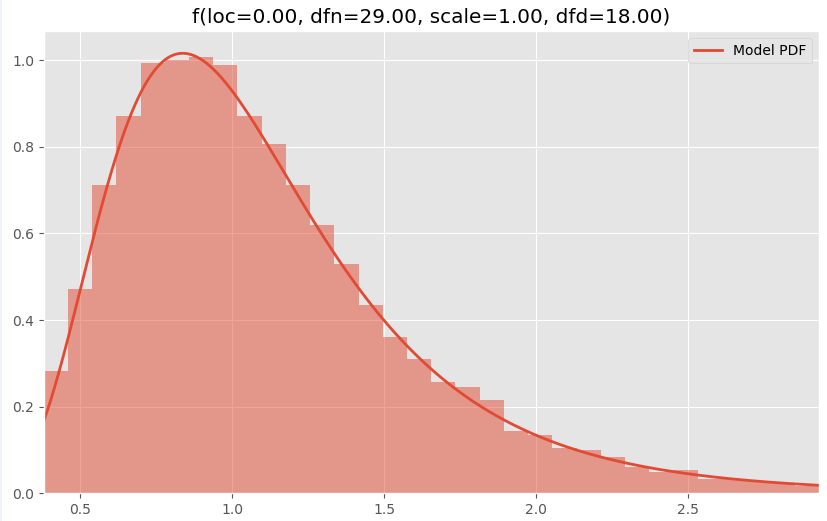基于这个思路出发，我又找出来了几个相似的分布曲线，对比如下：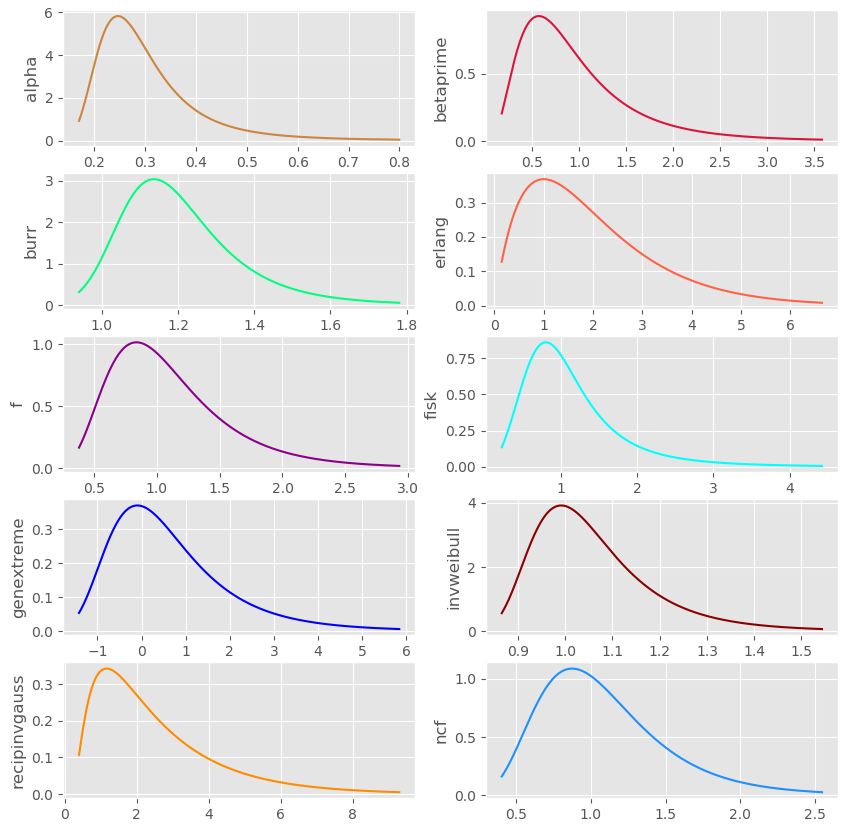上面的这些图的绘图风格都是使用了R里面ggplot的风格，换成我自己平时的风格如下：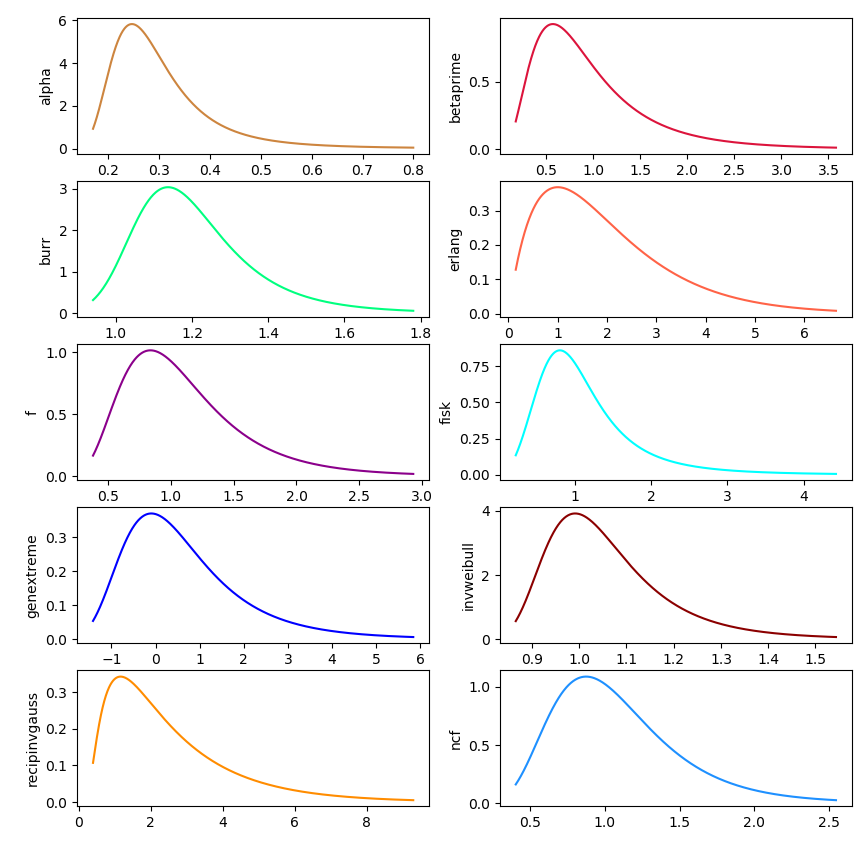个人还是比较喜欢下面的这种风格的，更为简洁清爽。

具体的实现代码如下：

#!usr/bin/env python
# encoding:utf-8
from __future__ import division

"""
__Author__:沂水寒城
功能： 基于scipy构建所需分布模型，可视化展示
"""

import matplotlib
import numpy as np
from scipy import stats
import matplotlib.pyplot as plt

#绘图设置
#matplotlib.style.use('ggplot')
color_list=['#CD853F','#DC143C','#00FF7F','#FF6347','#8B008B','#00FFFF','#0000FF','#8B0000','#FF8C00',
'#1E90FF','#00FF00','#FFD700','#008080','#008B8B','#8A2BE2','#228B22','#FA8072','#808080']

size=1000
distribution1=stats.alpha(a=3.57, loc=0.0, scale=1.0)
distribution2=stats.betaprime(a=5, b=6, loc=0.0, scale=1.0)
distribution3=stats.burr(c=10.5, d=4.3, loc=0.0, scale=1.0)
distribution4=stats.erlang(a=2, loc=0.0, scale=1.0)
distribution5=stats.f(dfn=29, dfd=18, loc=0.0, scale=1.0)
distribution6=stats.fisk(c=3.09, loc=0.0, scale=1.0)
distribution7=stats.genextreme(c=-0.1, loc=0.0, scale=1.0)
distribution8=stats.invweibull(c=10.6, loc=0.0, scale=1.0)
distribution9=stats.recipinvgauss(mu=0.63, loc=0.0, scale=1.0)
distribution10=stats.ncf(dfn=27, dfd=27, nc=0.416, loc=0.0, scale=1.0)
#分布模型集合
distribution_list=[distribution1,distribution2,distribution3,distribution4,distribution5,
distribution6,distribution7,distribution8,distribution9,distribution10]
label_list=['alpha','betaprime','burr','erlang','f','fisk','genextreme','invweibull',
'recipinvgauss','ncf']

plt.clf()
plt.figure(figsize=(10,30))
for i in range(len(distribution_list)):
plt.subplot(5,2,i+1)
distribution=distribution_list[i]
model_data=distribution.rvs(size=size)
x = np.linspace(distribution.ppf(0.01), distribution.ppf(0.99), size)
y = distribution.pdf(x)
plt.plot(x,y,c=color_list[i])
plt.ylabel(label_list[i])
plt.show()


感兴趣的话可以拿去实验，接下来就是依照具体的分布曲线来去一一去匹配或者是拟合原始的数据了。当然这里也可以通过显著性检验的形式来去做，在我之前的博客里面也有相关的说明和实现，感兴趣的话可以去看看。


展开全文• polyfit()1.2 非线性拟合 curve_fit()2 应用实例2.1 拟合活化能2.1.1 实验方法与原理2.1.2 结果与讨论2.1.3 附录：基于Python实现2.2 非理想反应器停留时间分布拟合2.2.1 引言2.2.2 研究方法2.2.3 结果与讨论 ...
目录1 非线性回归Python实现方法1.1 多项式拟合 polyfit()1.2 非线性拟合 curve_fit()2 应用实例2.1 拟合活化能2.1.1  实验方法与原理2.1.2  结果与讨论2.1.3 附录：基于Python实现2.2 非理想反应器停留时间分布拟合2.2.1    引言2.2.2  研究方法2.2.3  结果与讨论
1 非线性回归Python实现方法
在很多领域中往往需要对实验数据（或者调查数据）通过某种方程进行拟合，得到方程中的关键参数。在统计、物理、化学、生物等领域都是非常常见的方法。
非线性方程是指方程中存在非线性项，例如$e^x$，$\ln x$，$x^3$，$\sin x$等项时，求解过程通过人工计算很难得到准确的解。
1.1 多项式拟合 polyfit()
Python的numpy库中的函数polyfit()能够拟合一元多项式
$y = a_1 x^m + a_2 x^{m-1} +...+ a_m x + a_{m+1}$numpy.polyfit()专门用于拟合这种形式的多项式，看下面的例子
import numpy as np
import matplotlib.pyplot as plt

# 生成数据
x = np.linspace(0, 5, 100)
y = np.exp(x)
# 方差初始容器，辅助内容
i_range = [i for i in range(3,10)]
rsds = []
# 拟合并绘制不同项数多项式
for i in i_range:
p = np.polyfit(x, y, i, full=True)
y_hat = np.polyval(p, x)
rsds.append(p)
plt.plot(x, y_hat, lw=2, label=str(i))
# 绘制原始数据
plt.plot(x[::10], y[::10], 'r*', ms=20, label='exp(x)')
plt.legend()
# 字体大小设置
font = {'size':22}
plt.xlabel('x', font)
plt.ylabel('y', font)
plt.tick_params(labelsize=19)
plt.show()
# 绘制方差图
plt.plot(i_range, np.log10(rsds), '-o')
plt.xlabel('n', font)
plt.ylabel(r'$\lg \sigma^2$', font)
plt.tick_params(labelsize=19)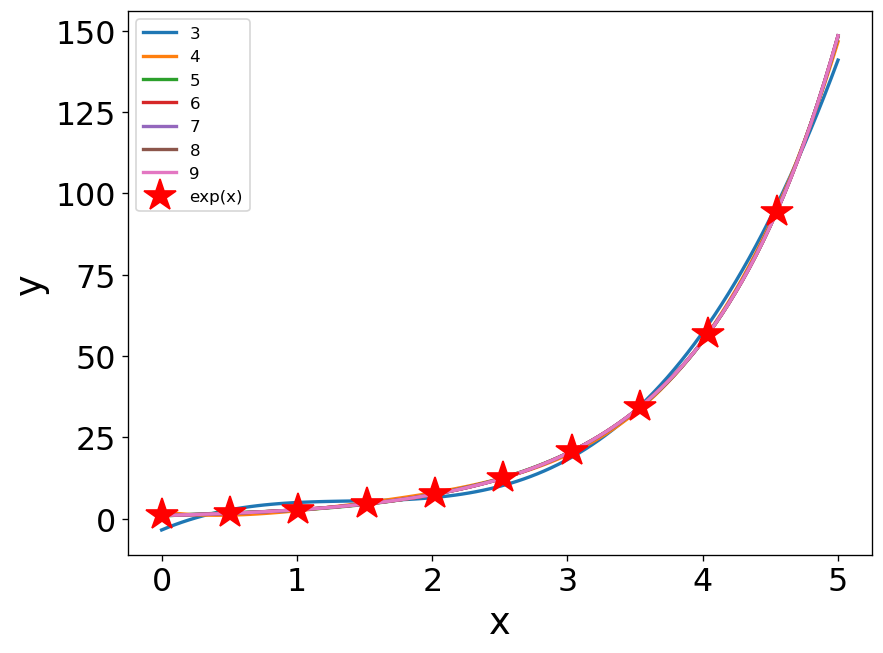图1-1多项式拟合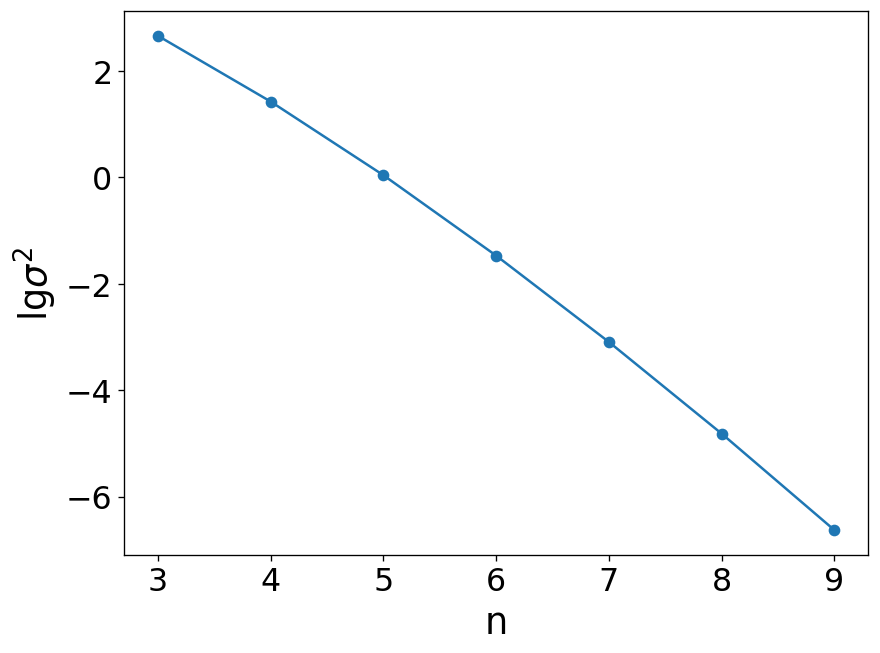图1-2 多项式拟合方差随多项式次数的变化规律
简单举个例子，$y=e^x$这个函数也可以拟合成多项式的形式，随着多项式次数的增加，拟合结果方差逐渐减小。其原理是连续函数的泰勒公式，高数里证明了连续可导的函数总能表示成多项式的形式。
多项式拟合能在一定程度上获得变化规律，但是也存在着无法忽略的问题，超出原始数据范围的预测效果差，而且随着n的增加，有可能出现过拟合的情况（噪音信号被带入拟合结果）。

1.2 非线性拟合 curve_fit()
curve_fit()是scipy.optimize的函数，用于拟合已知形式的函数，接下来还是看个例子。仍然以$y=\exp(x)$为例说明，通过随机数函数增加生成一些噪音，使噪音范围为[-0.2, 0.2），然后分别用curve_fit()和polyfit()拟合并对比。用下面的函数拟合，a和n为待拟合参数。
$y = a\exp(nx)$
代码如下：
from scipy.optimize import curve_fit
import numpy as np
import matplotlib.pyplot as plt
from random import random

# 生成数据
x = np.linspace(0, 5, 100)
y = np.exp(x)
# 随机函数生成噪音
eps = 0.4*np.array([random() for i in range(len(x))]) - 0.2
y = y * (1 + eps)

# 拟合函数形式
def f(x, a, n):
return a*np.exp(n*x)

# 非线性拟合
popt,pcov = curve_fit(f, x, y)
y_hat_c = f(x, popt, popt)
# 多项式拟合
p = np.polyfit(x, y, 6)
y_hat_p = np.polyval(p, x)
# 绘制图像
plt.plot(x, y, 'o', label='y')
plt.plot(x, y_hat_c, 'r', lw=2, label='curve_fit')
plt.plot(x, y_hat_p, 'k--', lw=2, label='polyfit_6')
# 设置字体
font = {'size':22}
plt.xlabel('x', font)
plt.ylabel('y', font)
plt.tick_params(labelsize=19)
plt.legend(fontsize=20)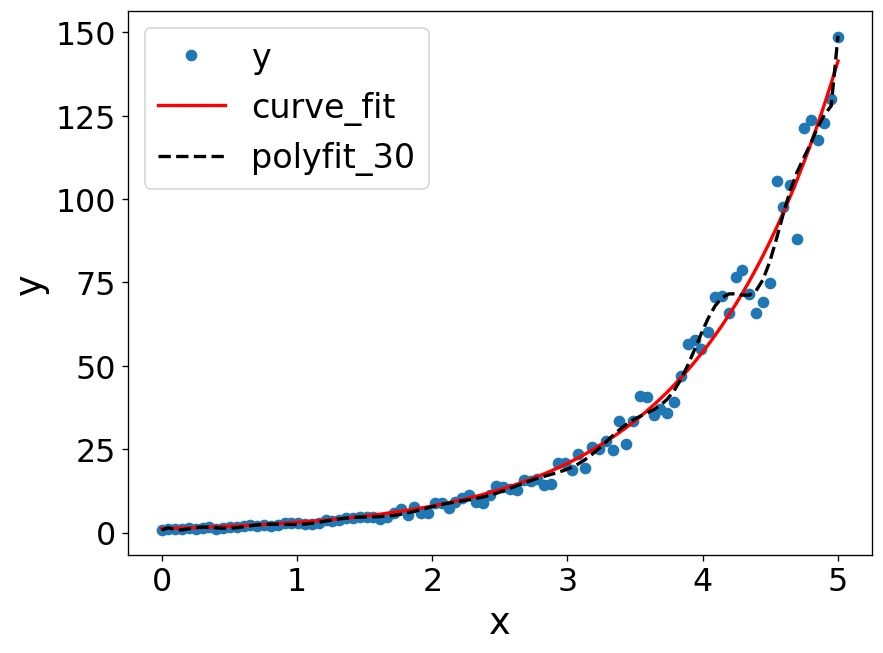图1-3 非线性拟合+多项式拟合过拟合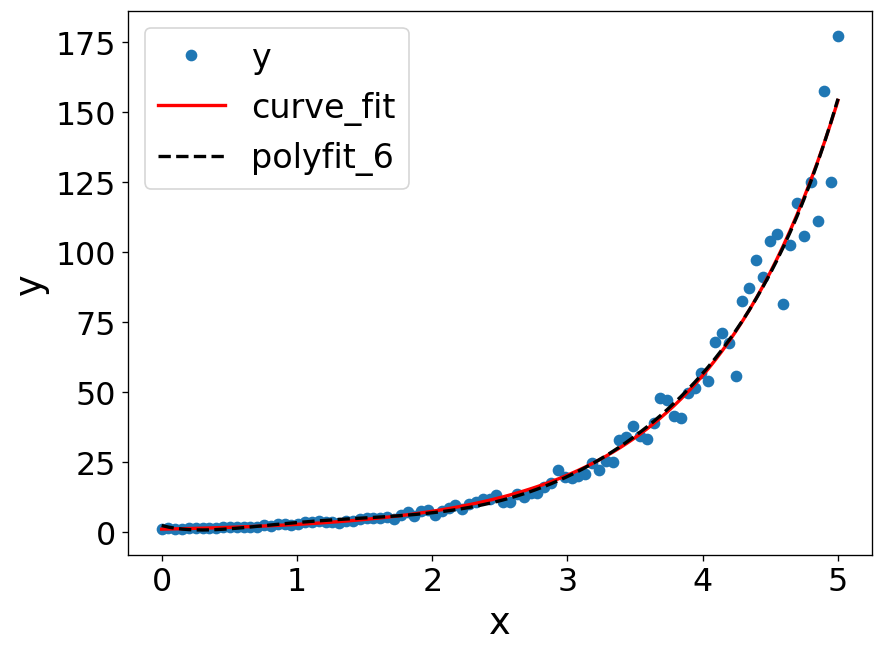图1-4 非线性拟合
拟合的结果是a=0.9663855 , n=1.01433087，和预期的1, 1很接近，拟合效果还可以。多项式拟合当使用30次方时，就产生了过拟合的情况，偏离了实际的情况，所以多项式拟合还是要慎用，次数取小了误差大，取大了会过拟合。

2 应用实例
2.1 拟合活化能
2.1.1  实验方法与原理
对于光催化反应甲醛反应，其动力学方程可表示为
$r = \frac{dc_A}{dt} = k_0\exp(-\frac{E}{RT})c_A^m$
分离变量后得
$\frac{dc_A}{c_A^m} = k_0\exp(-\frac{E}{RT})$
根据m取值的不同，浓度与时间的变化规律可分为三种形式的函数
$c_A(t) = c_0 - k_0\exp(-\frac{E}{RT})t,\ m=0\\ \ln c_A(t) = \ln c_0 - k_0\exp(-\frac{E}{RT})t,\ m=1\\ [c_A(t)]^{1-m} = c_0^{1-m} - (1-m)k_0\exp(-\frac{E}{RT})t,\ m\neq 0,1$
通过实验测得浓度随时间的变化规律cA(t)，根据不同的动力学模型对数据进行拟合。图2-1 实验浓度变化
2.1.2  结果与讨论
从实验数据初步判断m≠0，接下来将使用模型2和模型3对实验数据进行拟合。
$\ln r = m\ln c_0 + \ln k_0 - E/RT$
反应速率的表达式更为统一，对反应速率方程取对数后得到的方程形式更简洁，因此为减少在拟合过程中不必要的误差，可使用速率方程进行拟合，计算过程见附录，拟合结果如下。图2-2 速率与浓度变化规律
拟合得到m=1.75978，k0=24.8696，E=15949.7。拟合曲线与实验数据的对比如下图所示。图2-3 浓度随时间变化规律
通过非线性回归方法计算得出甲醛光催化反应的反应级数为1.76，活化能为15.9 kJ/mol。
2.1.3 附录：基于Python实现
import numpy as np
import matplotlib.pyplot as plt
from scipy.optimize import curve_fit

# 实验数据
t = [0,10,15,25,35,45,105,115,125,150,165,180,190,210,225,240,255,270]
c = [0.29, 0.26, 0.23, 0.2, 0.18, 0.16, 0.11, 0.1, 0.09, 0.09,
0.07, 0.06, 0.06, 0.06, 0.06, 0.06, 0.06, 0.06]
# 转换为array
t = np.array(t[:-1])
c = np.array(c[:-1])
# 反应速率
r = -np.diff(c)/np.diff(t)
T = 298

def mfun(c, m, k0, E):
''' 速率方程'''
lnr = m*np.log(c) + np.log(k0) - E/2477.6
return np.exp(lnr)

def mfun2(t, k0, E, m):
'''浓度方程，对于模型3'''
cm = 0.29**(1-m) - (1-m)*k0*np.exp(-E/2477.6)*t
return cm # 返回值为 c**(1-m)

def plot(x1, y1, x2, y2):
'''绘制速率-浓度图'''
font = {'size':22}
plt.plot(x1, y1, 'o')
plt.plot(x2, y2)
#plt.ylim([0,0.35])
plt.xlabel('c / ppm',font)
plt.ylabel('r / (ppm/s)',font)
plt.tick_params(labelsize=19)
plt.show()

def plot2(t1, c1, popt):
'''绘制浓度-时间图'''
# 生成数据
font = {'size':22}
t = np.linspace(t1,t1[-1],100)
cm = mfun2(t, popt, popt, popt)**(1/(1-popt))
# 绘图
plt.plot(t1, c1, 'o')
plt.plot(t, cm)
plt.ylim([0,0.35])
plt.xlabel('t / sec',font)
plt.ylabel('c(t) / ppm',font)
plt.tick_params(labelsize=19)

# 拟合数据，绘制图像
t1 = t[:-1]
p1,pcov1 = curve_fit(mfun, c[:-1], r) # very important
cf = np.linspace(c,c[-1],100) # 细化数据点
rf = mfun(cf, p1, p1, p1) # 计算拟合曲线
plot(c[:-1], r, cf, rf) # 绘制速率浓度图
plot2(t,c,p1) # 绘制浓度时间图


2.2 非理想反应器停留时间分布拟合
2.2.1    引言
非理想反应器内流体存在返混现象，因此流体微元在反应器中的停留时间并不是完全相同的，而存在与反应器特性有关的概率分布，称为停留时间分布。停留时间分布对于认识反应器传质过程与评估反应器生产能力等都有重要参考价值。
停留时间分布的测试方法分为两类：阶跃法和脉冲法。阶跃法是指在某一时刻突然改变稳定运行的反应器入口的探针物质浓度，通过监测不同时间后出口处探针物质浓度的变化规律，直接得到离散的停留时间分布F(t)。脉冲法是指在某一瞬间，从入口注入一定量探针物质，通过监测出口浓度，直接得到停留时间分布密度E(t)。图3-1 阶跃法停留时间分布示意图
停留时间分布函数F(t)与停留时间分布密度函数E(t)体现了反应器的某些工作特点，比如返混程度、平均停留时间等等。对于理想平推流反应器无返混，而理想全混釜反应器返混无穷大，通常非理想反应器的返混程度则是在两者之间。可见停留时间分布函数对于评估反应器生产能力、设计反应器体积等极为重要。图3-2 停留时间分布函数
2.2.2  研究方法
停留时间分布规律通常呈“S”型曲线，F(t)的最大值为1，因此可使用S型函数表示F(t)，其中b，c为拟合参数。将实验数据通过S函数拟合得到停留时间分布的连续函数。
$F(t) = \frac{1}{1+b\exp(-ct)}$
因此，进一步可解算停留时间分布密度函数E(t)，其形式可表示为如下方程：
$E(t) = \frac{dF(t)}{dt} = \frac{bc\exp(-ct)}{(1+b\exp(-ct))^2}$
平均停留时间则可表示为如下：
$\bar t = \int_0^\infty tE(t) dt = \frac{\ln (b+1)}{c}$
停留时间分布的方差表示为：
$\sigma^2_t = \int_0^\infty (t-\bar t)^2E(t)dt = \int_0^\infty t^2E(t)dt - \bar t^2$
2.2.3  结果与讨论
表 1 阶跃法测定停留时间分布实验数据

t/s
0
15
25
35
45
55
65
75
85
95

Cout/(kg m-3)
0
0.5
1.0
2.0
4.0
5.5
6.5
7.0
7.7
7.7

（1）通过离散方法计算得到=50.58，σt2=341.54。
（2）通过拟合S型函数得到停留时间分布函数：
$F(t) = \frac{1}{1+63.5564\exp(-0.0916t)}$
停留时间分布密度函数：
$E(t) = \frac{5.8218\exp(-0.0916t)}{(1+63.5564\exp(-0.0916t))^2}$
平均停留时间：
$\bar t = \frac{\ln (63.5564+1)}{0.0916} = 45.50$
停留时间分布方差：
$\sigma_t^2 = 364.60$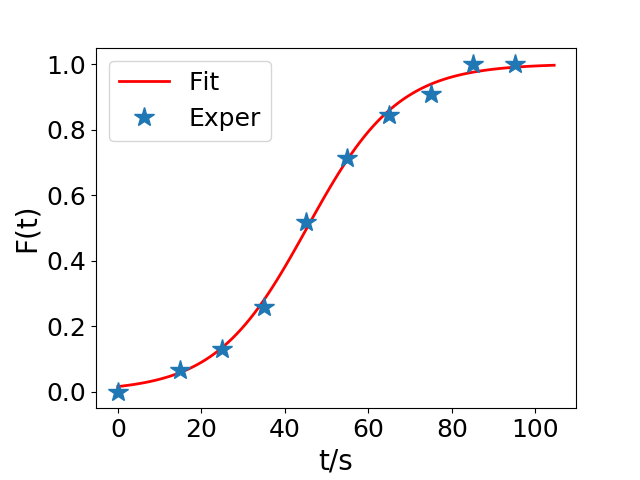图3-3 拟合停留时间分布函数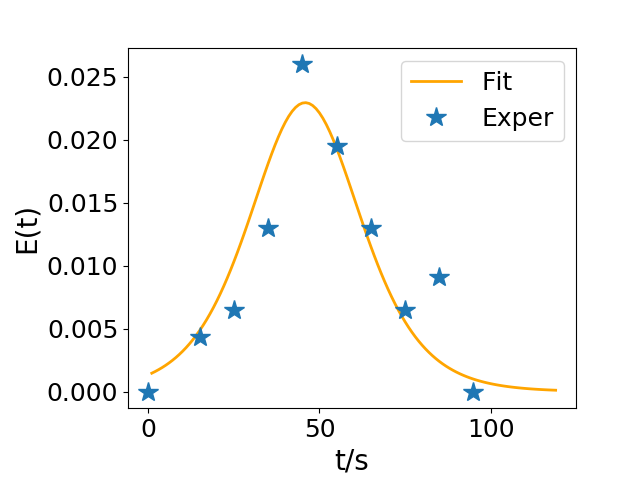*图3-4 拟合停留时间分布密度函数
拟合函数的计算结果与离散方法的计算结果相比，数值相差不超过10%，通过图像可以看到，S函数能较好的拟合出停留时间分布函数。经过多次尝试发现，S函数拟合返混程度较大的非理想反应器时效果较好，而对接近平推流的反应器拟合效果差。


展开全文• 如果你在寻找卡方分布是什么？如何实现卡方检验？那么请看这篇博客，将以通俗易懂的语言，全面的阐述卡方、卡方检验及其python实现。 1. 卡方分布 1.1 简介 ...${\chi ^{\text{2}}}$分布在数理统计...
如果你在寻找卡方分布是什么？如何实现卡方检验？那么请看这篇博客，将以通俗易懂的语言，全面的阐述卡方、卡方检验及其python实现。
1. 卡方分布
1.1 简介
抽样分布有三大应用：T分布、卡方分布和$\Gamma$分布。可以简单用四个字概括它们的作用：“以小博大”，即通过小数量的样本容量去预估总体容量的分布情况。这里开始介绍卡方分布。${\chi ^{\text{2}}}$分布在数理统计中具有重要意义。  ${\chi ^{\text{2}}}$分布是由阿贝(Abbe)于1863年首先提出的，后来由海尔墨特(Hermert)和现代统计学的奠基人之一的卡·皮尔逊(C K．Pearson)分别于1875年和1900年推导出来，是统计学中的一个非常有用的著名分布。
1.2 定义
若n个相互独立的随机变量${\xi _1},{\xi _2}, \cdots ,{\xi _n}$，均服从标准正态分布，则这n个服从标准正态分布的随机变量的平方和构成一新的随机变量${\text{X}}\left( {} \right)$，其分布规律称为卡方分布。记作：
$${\text{X}} \sim {\chi ^{\text{2}}}\left( k \right)$$
1.3 卡方分布的密度函数
$${f_k}(x) = \frac{{{{(1/2)}^{\frac{k}{2}}}}}{{\Gamma (k/2)}}{x^{\frac{k}{2} - 1}}{e^{ - \frac{x}{2}}}$$卡方分布的概率密度图
其中x≥0, 当x≤0时fk(x) = 0。这里$\Gamma$代表Gamma 函数。
在大多数涉及卡方分布的书中都会提供它的累积分布函数的对照表。此外许多表格计算软件如OpenOffice.org Calc和Microsoft Excel中都包括卡方分布函数。
卡方分布可以用来测试随机变量之间是否相互独立，也可用来检测统计模型是否符合实际要求。自由度为 k 的卡方变量的平均值是 k，方差是 2k。

1.4 性质（后续填坑）2. 卡方检验
2.1 简介
卡方检验(chi-square，记为χ2\chi^2检验)是统计学中常用来计数数据分析的方法，对于总体的分布不作任何假设，因此它属于非参数检验法中的一种。本博文从理论到实际应用去阐述卡方检验，最后用python语言去实现卡方分布的代码。
${\chi ^{\text{2}}}$检验的基本思想是根据样本数据推断总体的频次与期望频次有显著性差异， ${\chi ^{\text{2}}}$的计算公式为：
$${\chi ^{\text{2}}} = \frac{{{{({f_0} - {f_e})}^2}}}{{{f_e}}}$$
其中，${{f_0}}$为实际观察频次，${{f_e}}$为理论值
这是卡方检验的原始公式，其中当${{f_e}}$越大，近似效果越好。显然${{f_0}}$与${{f_e}}$相差越大，卡方值就越大；${{f_0}}$与${{f_e}}$相差越小，卡方值就越小。因此它能够用来表示${{f_0}}$与${{f_e}}$相差的程度。根据这个公式，可认为卡方检验的一般问题是要检验名义型变量的实际观测次数和理论次数分布之间是否存在显著差异。
一般卡方检验方法进行统计检验时，要求样本容量不宜太小，理论次数$\geqslant 5$，否则需要进行校正。如果个别单元格的理论次数小于5，处理的方法有以下四种：
单元格合并法
增加样本数
去除样本数
使用校正公式，当某一期次数小于5时，应该利用校正公式计算卡方值。校正公式为：$${\chi ^{\text{2}}} = \sum {\frac{{{{(\left| {{f_0} - {f_e}} \right| - 0.5)}^2}}}{{{f_e}}}}$$
知道了卡方分布的原理，那具体是如何使用的呢？卡方分析有两个常见的应用——适合度(拟合性)分析和独立性分析。从我目前的经验来看，这也是应用十分广泛的一种统计分析方式。那么什么是卡方适合度分析和独立性分析呢？且听我慢慢道来。
“适配度检验”验证一组观察值的次数分配是否异于理论上的分配。
2.2 卡方独立性检验
“独立性检验”验证从两个变量抽出的配对观察值组是否互相独立（例如：每次都从A国和B国各抽一个人，看他们的反应是否与国籍无关）。
独立性检验主要用于两个或两个以上因素多项分类的计数资料分析，也就是研究两类变量之间的关联性和依存性问题。如果两变量无关联即相互独立，说明对于其中一个变量而言，另一变量多项分类次数上的变化是在无差范围之内；如果两变量有关联即不独立，说明二者之间有交互作用存在。
独立性检验一般采用列联表的形式记录观察数据, 列联表是由两个以上的变量进行交叉分类的频数分布表，是用于提供基本调查结果的最常用形式，可以清楚地表示定类变量之间是否相互关联。又可具体分为：
四格表的独立性检验：又称为2*2列联表的卡方检验。四格表资料的独立性检验用于进行两个率或两个构成比的比较，是列联表的一种最简单的形式。
行x列表资料的独立性检验：又称为RxC列联表的卡方检验。行x列表资料的独立性检验用于多个率或多个构成比的比较
2.3.1 独立性检验步骤
Step1：建议原假设
H0：两变量相互独立；H1：两变量相互不独立
Step2：计算自由度与理论频数
自由度：$df = (r - 1)(c - 1)$，理论频数：${e_{ij}} = \frac{{{F_{{Y_i}}} \cdot {F_{{X_j}}}}}{n}$
Step3：计算统计量
$${\chi ^{\text{2}}} = \sum\limits_{i = 1}^r {\sum\limits_{j = 1}^c {\frac{{{{({f_{ij}} - {e_{ij}})}^2}}}{{{e_{ij}}}}} } \sim {\chi ^{\text{2}}}(df)$$
Step4：查${\chi ^{\text{2}}}$分布临界值表，确定接受域和拒绝域
接受域：$${\chi ^{\text{2}}}_{1 - \frac{\alpha }{2}}(df) < {\chi ^{\text{2}}} < {\chi ^{\text{2}}}_{\frac{\alpha }{2}}(df)$$
2.3.2 应用条件
要求样本含量应大于40且每个格子中的理论频数不应小于5。当样本含量大于40但理论频数有小于5的情况时卡方值需要校正，即公式：$${\chi ^{\text{2}}} = \sum {\frac{{{{(\left| {{f_0} - {f_e}} \right| - 0.5)}^2}}}{{{f_e}}}}$$
2.3.3 应用实例
例：对下表所示频数分布表，以95%显著水平，检验色觉与性别是否有关。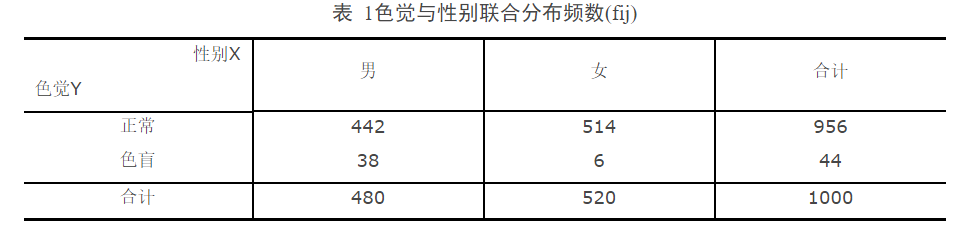解：
Step1：H0：色觉与性别相互独立；H1：色觉与性别相互不独立（相关）
Step2：自由度$df = (r - 1)(c - 1) = (2 - 1)(2 - 1) = 1$，理论频数计算如表2。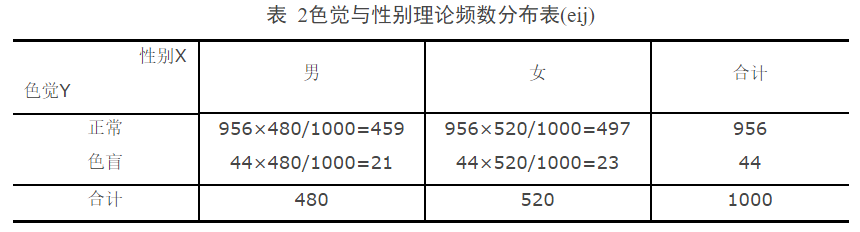Step3：计算统计量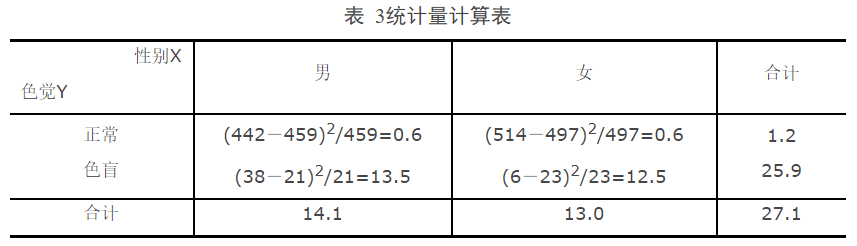Step4：查分布临界值表
$${\chi ^{\text{2}}}_{0.975}(1) = 0.00098,{\chi ^{\text{2}}}_{0.025}(1) = 5.0239$$
统计推断：${\chi ^{\text{2}}} = 27.1 > 5.0239$，所以拒绝原假设，即色觉与性别相互不独立。
2.3.4 RxC列联表的卡方检验
专用公式：
r行c列表资料卡方检验的${\chi ^{\text{2}}} = n \times (\frac{{{A_{11}}}}{{{n_1}{n_1}}} + \frac{{{A_{12}}}}{{{n_1}{n_2}}} + \cdots + \frac{{{A_{rc}}}}{{{n_r}{n_c}}}) - 1$
应用条件

要求每个格子中的理论频数T均大于5或1<T<5的格子数不超过总格子数的1/5。当有T<1或1<T<5的格子较多时，可采用并行并列、删行删列、增大样本含量的办法使其符合行x列表资料卡方检验的应用条件。多个率的两两比较可采用行x列表分割的办法。
独立性检验的理论频数的计算公式为：
$${f_e} = \frac{{{f_{xi}} \times {f_{yi}}}}{N}$$
2.3.5 独立性检验python实现
（1）环境配置

1 # -*- coding:utf-8 -*-
2 # @Time    : 2018/6/29 18:08
3 # @Author  : yuanjing liu
4 # @Email   : lauyuanjing@163.com
5 # @File    : 4.py
6 # @Software: PyCharm
7
8 # 卡方独立性检验
9 import numpy as np
10 from scipy.stats import chi2_contingency
11 from scipy.stats import chi2

（2）卡方独立性检验函数

1 '''
2 （1）假设检验重要知识
3 H0:A与B相互独立  H1：A与B不相互独立
4 若卡方值大于临界值，拒绝原假设，表示A与B不相互独立，A与B相关
5 函数中re返回为1表示拒绝原假设，0表示接受原假设
6
7 （2）参数说明
8 输入：
9 alpha --- 置信度，用来确定临界值
10 data  --- 数据，请使用numpy.array数组
11 输出：
12 g     --- 卡方值，也就是统计量
13 p     --- P值（统计学名词），与置信度对比，也可进行假设检验，P值小于置信度，即可拒绝原假设
14 dof   --- 自由度
15 re    --- 判读变量，1表示拒绝原假设，0表示接受原假设
16 expctd--- 原数据数组同维度的对应理论值
17
18 （3）应用场景
19 要求样本含量应大于40且每个格子中的理论频数不应小于5
20
21 理论知识详见博客：
22 '''
23
24
25 def chi2_independence(alpha, data):
26     g, p, dof, expctd = chi2_contingency(data)
27
28     if dof == 0:
29         print('自由度应该大于等于1')
30     elif dof == 1:
31         cv = chi2.isf(alpha * 0.5, dof)
32     else:
33         cv = chi2.isf(alpha * 0.5, dof-1)
34
35
36     if g > cv:
37         re = 1  # 表示拒绝原假设
38     else:
39         re = 0  # 表示接受原假设
40
41     return g, p, dof, re, expctd

（3）测试

1 # test
2 alpha1 = 0.05  # 置信度，常用0.01，0.05，用于确定拒绝域的临界值
3 data1 = np.array([[442, 514], [38, 6]])
4 g, p, dof, re, expctd = chi2_independence(alpha1, data1)

（4）输出

1 g = 25.554809338151387
2 p = 4.2999294019586401e-07
3 dof = 1
4 re = 1
5 expctd = array([[ 458.88,  497.12], [  21.12,   22.88]])

输出变量的含义，在函数处都已经说明，re=1表示拒绝原假设，结果和上面例子手算的一致。

2.4 卡方拟合性检验
2.4.1 定义
卡方检验能检验单个多项分类名义型变量各分类间的实际观测次数与理论次数之间是否一致的问题，这里的观测次数是根据样本数据得多的实计数，理论次数则是根据理论或经验得到的期望次数。这一类检验称为拟合性检验。其自由度通常为分类数减去1，理论次数通常根据某种经验或理论。
总而言之，卡方拟合度检验用于判断不同类型结果的比例分布相对于一个期望分布的拟合程度。
2.4.2 应用条件
卡方拟合性检验适用于变量为类别型变量的情况。 例如：变量为有罪或无罪。
当每个类别中观察到的或预期的频率太小时，此检验无效。要求样本含量应大于40且每个格子中的理论频数不应小于5
2.4.3 应用实例
随机抽取60名高一学生，问他们文理要不要分科，回答赞成的39人，反对的21人，问对分科的意见是否有显著的差异。
解：
（1）提出H0：学生们对文理分科的意见没有差异
（2）分析：如果没有显著的差异，则赞成与反对的各占一般，因此是一个无差假设的检验，于是理论次数为60/2=30，带入公式：
$${\chi ^{\text{2}}}{\text{ = }}\sum {\frac{{{{\left( {{f_0} - {f_e}} \right)}^2}}}{{{f_e}}}} = \frac{{{{\left( {39 - 30} \right)}^2}}}{{30}} + \frac{{{{\left( {21 - 30} \right)}^2}}}{{30}} = 5.4 > \chi _{0.05}^2\left( 1 \right) = 3.84$$
其中自由度df = 类别-1 = 2-1 = 1
所以拒绝原假设，认为对于文理分科，学生们的态度是有显著的差异的。
2.4.4 卡方拟合性检验python实现
（1）环境配置

1 # 卡方拟合性检验
2 import numpy as np
3 from scipy.stats import chisquare
4 from scipy.stats import chi2

（2）卡方独立性检验函数

1 '''
2 （1）假设检验重要知识
3 H0:类别A与B的比例没有差异  H1：类别A与B的比例有差异
4 若卡方值大于临界值，拒绝原假设，表示A与B不相互独立，A与B相关
5 函数中re返回为1表示拒绝原假设，0表示接受原假设
6
7 （2）参数说明
8 输入：
9 alpha --- 置信度，用来确定临界值
10 data  --- 数据，请使用numpy.array数组
11 sp  --- 表示输入数组的形状参数，默认为一维
12 输出：
13 chis     --- 卡方值，也就是统计量
14 p_value  --- P值（统计学名词），与置信度对比，也可进行假设检验，P值小于置信度，即可拒绝原假设
15 cv --- 拒绝域临界值
16 j   --- 自由度
17 re    --- 判读变量，1表示拒绝原假设，0表示接受原假设
18
19 （3）应用场景
20 要求样本含量应大于40且每个格子中的理论频数不应小于5
21
22 理论知识详见博客：
23 '''
24
25
26 def chi2_fitting(data, alpha, sp=None):
27     chis, p_value = chisquare(data, axis=sp)
28     i, j = data.shape  # j为自由度
29
30     if j == 0:
31         print('自由度应该大于等于1')
32     elif j == 1:
33         cv = chi2.isf(alpha * 0.5, j)
34     else:
35         cv = chi2.isf(alpha * 0.5, j - 1)
36
37     if chis > cv:
38         re = 1  # 表示拒绝原假设
39     else:
40         re = 0  # 表示接受原假设
41
42     return chis, p_value, cv, j-1, re

（3）测试

1 data1 = np.array([[39, 21], ])
2 alpha1 = 0.05
3 chis1, p_value1, cv1, dof, re1 = chi2_fitting(data1, alpha1)

（4）输出

chis1 = 5.4000000000000004
p_value1 = 0.020136751550346329
cv1 = 5.0238861873148917
dof = 1
re1 = 1

显然chis1(卡方值)是大于cv1(临界值)的，因此拒绝原假设，认为对于文理分科，学生们的态度是有显著的差异的。与前面结果一直。这里可以输入多维度数组，而不是简单的一维数组，因为在国内没有找到合适的多维度多类别数组的例子，想结合例子来讲解，更便于理解，既然没有例子也就不使用python进行这个知识点的展示了，望理解。但是此函数中sp给多维度多类别的数组输入提供了方法，原理和scipy.stats.chisquare中的axis参数一样，请大家查阅官方文档。

2.5 卡方拟合性检验和独立性检验之间的关系
从表面上看，拟合性检验和独立性检验不论在列联表的形式上，还是在计算卡方的公式上都是相同的，所以经常被笼统地称为卡方检验。但是两者还是存在差异的。
首先，两种检验抽取样本的方法不同。如果抽样是在各类别中分别进行，依照各类别分别计算其比例，属于拟合优度检验。如果抽样时并未事先分类，抽样后根据研究内容，把入选单位按两类变量进行分类，形成列联表，则是独立性检验。
其次，两种检验假设的内容有所差异。拟合优度检验的原假设通常是假设各类别总体比例等于某个期望概率，而独立性检验中原假设则假设两个变量之间独立。
最后，期望频数的计算不同。拟合优度检验是利用原假设中的期望概率，用观察频数乘以期望概率，直接得到期望频数。独立性检验中两个水平的联合概率是两个单独概率的乘积。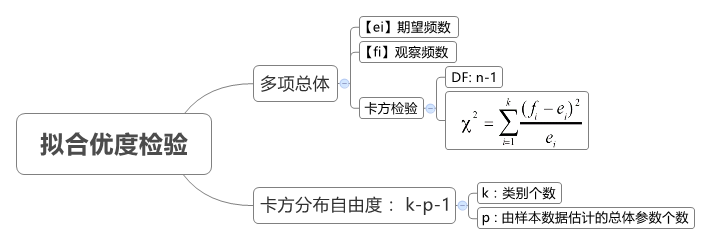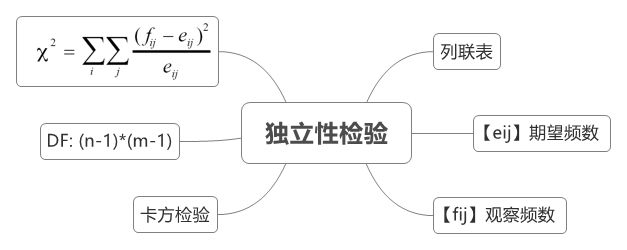3 文献
卡方检验 - 维基百科，自由的百科全书https://zh.wikipedia.org/wiki/%E5%8D%A1%E6%96%B9%E6%A3%80%E9%AA%8C
卡方分布 - MBA智库百科http://wiki.mbalib.com/wiki/%E5%8D%A1%E6%96%B9%E5%88%86%E5%B8%83
用Python进行卡方分析 - 简书https://www.jianshu.com/p/c307d04eee56
拟合优度检验_百度百科https://baike.baidu.com/item/%E6%8B%9F%E5%90%88%E4%BC%98%E5%BA%A6%E6%A3%80%E9%AA%8C
列联表分析2——独立性检验（卡方检验）_关文忠工作室_新浪博客http://blog.sina.com.cn/s/blog_4d69c7430101ndub.html
卡方分布分析与应用 - 云+社区 - 腾讯云https://cloud.tencent.com/developer/article/1010577
卡方检验 | Ren’s Cabinet of Curiositieshttp://www.ryanzhang.info/tag/%E5%8D%A1%E6%96%B9%E6%A3%80%E9%AA%8C/
scipy.stats.chi2 — SciPy v1.1.0 Reference Guide（卡方分布）https://docs.scipy.org/doc/scipy/reference/generated/scipy.stats.chi2.html#scipy.stats.chi2
scipy.stats.chi2_contingency — SciPy v0.15.1 Reference Guide（卡方独立性检验）https://docs.scipy.org/doc/scipy-0.15.1/reference/generated/scipy.stats.chi2_contingency.html
scipy.stats.chisquare — SciPy v1.1.0 Reference Guide（卡方拟合性检验）https://docs.scipy.org/doc/scipy/reference/generated/scipy.sta转载说明
1、本人博客纯属技术积累和分享，欢迎大家评论和交流以求共同进步。
2、在无明确说明下，博客可以转载以供个人学习和交流，但是要附上出处。
3、如果原创博客使用涉及商业/公司行为请邮件(1547364995@qq.com)告知，一般情况均会及时回复同意。
4、如果个人博客中涉及他人文章我会尽力注明出处，但受限于能力并不能保证所有引用之处均能够注明出处，如有冒犯，请您及时邮件告知以便修改，并于此提前向您道歉。
5、转载过程中如有涉及他人作品请您与作者联系。
6、所有文章（不限于原创）仅为个人见解，个人只能尽量保证正确，如有错误您需要自负责任，并请您留下评论提出错误之处以便及时更正，惠泽他人，谢谢

转载于:https://www.cnblogs.com/Yuanjing-Liu/p/9252844.html
展开全文• 其根本思想就是在于比较理论频数和实际频数的吻合程度或拟合优度问题。 卡方检验的基本思想： 卡方检验是以χ2\chi^2χ2分布为基础的一种常用假设检验方法，它的基本假设H0是：观察频数与期望频数没有差别。 该检验...
• 1. 从np.random.normal()到正态分布拟合https://blog.csdn.net/lanchunhui/article/details/50163669 matplotlib.pyplot.hist()转载：python中plt.hist参数详解 matplotlib tutorial 2. Introduction to the ...数据分析
• mix_T Scipy和sklearn目前没有任何资源可用于拟合... 使用类似于scikit-learn的界面将数据集拟合到多元Student T分布的有限混合并计算关键统计量（BIC，AIC等）； 使用变分近似将数据集拟合为多元学生T分布的混合。
• 文章目录1. 概率论基础1.1 概率公式... 最大似然估计5.1 过拟合 1. 概率论基础 1.1 概率公式 1.2 贝叶斯公式 1.3 分布 两点分布 二项分布(伯努利分布) 方法一: Taylor展开式 泊松分布 2. 统计...机器学习
• 对数据进行建模处理时，...'''输出结果中第一个为统计量，第二个为P值（统计量越接近1越表明数据和正态分布拟合的好， P值大于指定的显著性水平，接受原假设，认为样本来自服从正态分布的总体）''' print(stats.shap...
• 在统计学中，差异显著性检验是“统计假设检验”（Statistical hypothesis testing）的一种，用于检测科学实验中实验组与对照组之间是否有差异以及差异是否显著的办法。 在实验进行过程中，尽管尽量排除随机误差的...假设检验 非参数检验 Scipy 差异分析
• 朴素贝叶斯方法是基于贝叶斯...贝叶斯法对条件概率分布做了条件独立性假设，从而减少了模型的复杂性，增加了模型的泛化能力，减少了过拟合的风险。 #后验概率最大化 可以证明，期望风险最小化准则可以得到后验概率...
• MATLAB用拟合出的代码绘图 高斯处理一切 gpe是一个R包，用于实现高斯过程（GP）统计建模框架。 该项目的目的是提供其他语言提供的功能齐全的GP工具箱的某些功能，例如python和MATLAB。 尽管主要是关于将GP方法的功能...
• 决策树可以转换成一个if-then规则的集合，也可以看作是定义在特征空间划分上的类的条件概率分布。 2．决策树学习旨在构建一个与训练数据拟合很好，并且复杂度小的决策树。因为从可能的决策树中直接选取最优决策树是...
• 最后，通过大量统计分析和机器学习案例提供实践指南，首先讲解回归分析、区间分布、数据图形化、分布趋势、正态分布分布拟合等数据分析基础，然后讲解神经网络、统计算法、欧氏距离、余弦相似度、线性与非线性回归...
• Seaborn的displot()集合了matplotlib的hist()与核函数估计kdeplot的功能，增加了rugplot分布观测条显示与利用scipy库fit拟合参数分布的新颖用途。 seaborn.distplot(a, bins=None, hist=True, kde=True, rug=False, ...数据可视化 可视化 大数据
• CONNORAV 使用 Tuenter (2001) 描述的优化技术来实现这一点，以将这些统计数据拟合到分析性的 Johnson SU 分布。 虽然并非所有分布都可以单独使用均值、标准差、偏斜和峰度来描述，但很多数据都类似于这些形状，...
• 1.1 Python 简介......................................1 1.2 安装软件包......................................2 1.2.1 Python(x,y)..................................... 2 1.2.2 Enthought Python ...
• 将连续分布（例如GEVD，GPD或用户指定的连续分布拟合到提取的极端事件 可视化模型输入，结果和拟合优度统计 给定概率或返回期的极端事件（例如100年事件）和相应置信区间的估计 协助模型选择和调整的工具，例如BM...
• 散点图是指在数理统计回归分析中，数据点在直角坐标系平面上的分布图，散点图表示因变量随自变量而变化的大致趋势，由此趋势可以选择合适的函数进行经验分布拟合，进而找到变量之间的函数关系。 二、散点图有什么...
• 模型选择基本是选择一个模型评估指标（均方差、准确率、F1等）同时考虑欠拟合（在训练集上表现不好-为将训练样本的一般性质学好）和过拟合（在训练集和测试集上表现有很大差异-将训练集上的性质学习的过好）的综合...
• 介绍：利用观测数据判断总体是否服从正态分布的检验称为正态性检验，它是统计判决中重要的一种特殊的拟合优度假设检验。 方法：直方图初判 、 QQ图判断、 K-S检验 引入库 import matplotlib.pyplot as plt import ...
• PyMC3是一个高级Python库，用于使用概率编程构建统计模型，并使用现代贝叶斯计算方法进行拟合。 我将提供贝叶斯推理和预测的简介，然后是有关使用PyMC3进行概率编程的教程，包括使用真实数据集使用马尔可夫链...
• 权重参数分布中的不确定性是通过贝叶斯统计方法在出色的包的帮助下。 这项工作尚处于初期阶段，因此仍然缺少许多功能。 具有GAM功能的其他项目 目录 安装 该软件包可以在PyPi中找到。 pip install gammy 例子 '...
• 利用观测数据判断总体是否服从正态分布的检验称为正态性检验，它是统计判决中重要的一种特殊的拟合优度假设检验。 直方图初判 ：直方图 + 密度线 QQ图判断：(s_r.index – 0.5)/len(s_r) p(i)=(i-0.5)/n 分 位数与...
• 利用观测数据判断总体是否服从正态分布的检验称为正态性检验，它是统计判决中重要的一种特殊的拟合优度假设检验。 直方图初判 ：直方图 + 密度线   QQ图判断：(s_r.index - 0.5)/len(s_r) p(i)=(i-0.5)/n 分 ...
• 平均剩余寿命，分位数，描述性统计摘要，分布随机抽样 概率密度函数（PDF），累积分布函数（CDF），生存函数（SF），危害函数（HF）和累积危害函数（CHF）的图 轻松创建分发对象。 例如。 dist = Weibull_......

# python拟合统计分布python 订阅